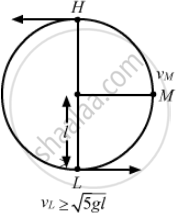Department of Pre-University Education, KarnatakaPUC Karnataka Science Class 11
Advertisement Remove all ads

# A Particle is Rotated in a Vertical Circle by Connecting It to a String of Length L And Keeping the Other End of the String Fixed. - Physics

MCQ

A particle is rotated in a vertical circle by connecting it to a string of length l and keeping the other end of the string fixed. The minimum speed of the particle when the string is horizontal for which the particle will complete the circle is

#### Options

• $\sqrt{gl}$

• $\sqrt{2gl}$

• $\sqrt{3gl}$

• $\sqrt{5gl}$

Advertisement Remove all ads

#### Solution

$\sqrt{3gl}$
Suppose that one end of an extensible string is attached to a mass m, while the other end is fixed. The mass moves with a velocity v in a vertical circle of radius R. At some instant, the string makes an angle θ with the vertical as shown in the figure.For a complete circle, the minimum velocity at L must be $v_L = \sqrt{5gl}$ .

Applying the law of conservation of energy, we have:
Total energy at M = total energy at L
$\text{ i .e } . , \frac{1}{2}m {v_M}^2 + mgl = \frac{1}{2}m {v_L}^2$
$\Rightarrow \frac{1}{2}m {v_M}^2 = \frac{1}{2}m {v_L}^2 - mgl$
$\text{ Using } v_L \geq \sqrt{5gl}, \text{ we have} :$
$\frac{1}{2}m {v_M}^2 \geq \frac{1}{2}m(5gl) - mgl$
$\therefore v_M = \sqrt{3gl}$

Is there an error in this question or solution?
Advertisement Remove all ads

#### APPEARS IN

HC Verma Class 11, 12 Concepts of Physics 1
Chapter 8 Work and Energy
MCQ | Q 10 | Page 131
Advertisement Remove all ads
Advertisement Remove all ads
Share
Notifications

View all notifications

Forgot password?